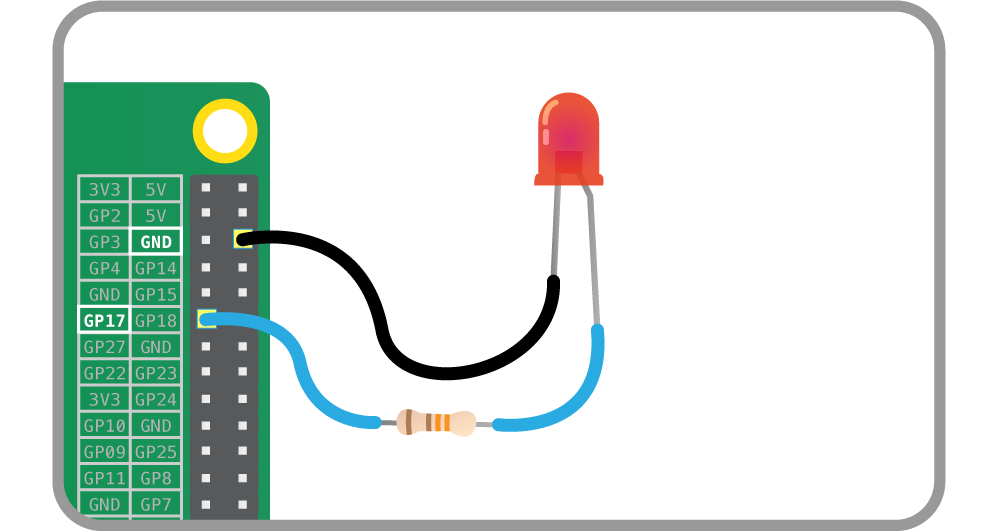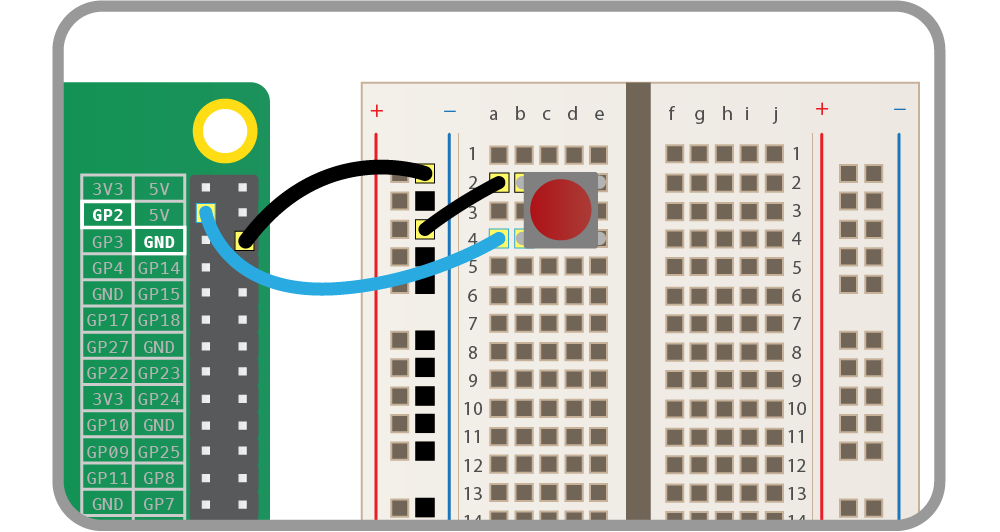# Recipes¶

The following recipes demonstrate some of the capabilities of the gpiozero library. Please note that all recipes are written assuming Python 3. Recipes may work under Python 2, but no guarantees!

## Pin Numbering¶

This library uses Broadcom (BCM) pin numbering for the GPIO pins, as opposed to physical (BOARD) numbering. Unlike in the RPi.GPIO library, this is not configurable.

Any pin marked `GPIO` in the diagram below can be used for generic components:## LED¶Turn an `LED` on and off repeatedly:

```from gpiozero import LED
from time import sleep

red = LED(17)

while True:
red.on()
sleep(1)
red.off()
sleep(1)
```

Alternatively:

```from gpiozero import LED
from signal import pause

red = LED(17)

pause()
```

Note

Reaching the end of a Python script will terminate the process and GPIOs may be reset. Keep your script alive with `signal.pause()`. See Keep your script running for more information.

## Button¶Check if a `Button` is pressed:

```from gpiozero import Button

button = Button(2)

while True:
if button.is_pressed:
print("Button is pressed")
else:
print("Button is not pressed")
```

Wait for a button to be pressed before continuing:

```from gpiozero import Button

button = Button(2)

button.wait_for_press()
print("Button was pressed")
```

Run a function every time the button is pressed:

```from gpiozero import Button
from signal import pause

def say_hello():
print("Hello!")

button = Button(2)

button.when_pressed = say_hello

pause()
```

## Button controlled LED¶Turn on an `LED` when a `Button` is pressed:

```from gpiozero import LED, Button
from signal import pause

led = LED(17)
button = Button(2)

button.when_pressed = led.on
button.when_released = led.off

pause()
```

Alternatively:

```from gpiozero import LED, Button
from signal import pause

led = LED(17)
button = Button(2)

led.source = button.values

pause()
```

## Traffic Lights¶A full traffic lights system.

Using a `TrafficLights` kit like Pi-Stop:

```from gpiozero import TrafficLights
from time import sleep

lights = TrafficLights(2, 3, 4)

lights.green.on()

while True:
sleep(10)
lights.green.off()
lights.amber.on()
sleep(1)
lights.amber.off()
lights.red.on()
sleep(10)
lights.amber.on()
sleep(1)
lights.green.on()
lights.amber.off()
lights.red.off()
```

Alternatively:

```from gpiozero import TrafficLights
from time import sleep
from signal import pause

lights = TrafficLights(2, 3, 4)

def traffic_light_sequence():
while True:
yield (0, 0, 1) # green
sleep(10)
yield (0, 1, 0) # amber
sleep(1)
yield (1, 0, 0) # red
sleep(10)
yield (1, 1, 0) # red+amber
sleep(1)

lights.source = traffic_light_sequence()

pause()
```

Using `LED` components:

```from gpiozero import LED
from time import sleep

red = LED(2)
amber = LED(3)
green = LED(4)

green.on()
amber.off()
red.off()

while True:
sleep(10)
green.off()
amber.on()
sleep(1)
amber.off()
red.on()
sleep(10)
amber.on()
sleep(1)
green.on()
amber.off()
red.off()
```

## Push button stop motion¶

Capture a picture with the camera module every time a button is pressed:

```from gpiozero import Button
from picamera import PiCamera

button = Button(2)

with PiCamera() as camera:
camera.start_preview()
frame = 1
while True:
button.wait_for_press()
camera.capture('/home/pi/frame%03d.jpg' % frame)
frame += 1
```

See Push Button Stop Motion for a full resource.

## Reaction Game¶When you see the light come on, the first person to press their button wins!

```from gpiozero import Button, LED
from time import sleep
import random

led = LED(17)

player_1 = Button(2)
player_2 = Button(3)

time = random.uniform(5, 10)
sleep(time)
led.on()

while True:
if player_1.is_pressed:
print("Player 1 wins!")
break
if player_2.is_pressed:
print("Player 2 wins!")
break

led.off()
```

See Quick Reaction Game for a full resource.

## GPIO Music Box¶

Each button plays a different sound!

```from gpiozero import Button
import pygame.mixer
from pygame.mixer import Sound
from signal import pause

pygame.mixer.init()

sound_pins = {
2: Sound("samples/drum_tom_mid_hard.wav"),
3: Sound("samples/drum_cymbal_open.wav"),
}

buttons = [Button(pin) for pin in sound_pins]
for button in buttons:
sound = sound_pins[button.pin.number]
button.when_pressed = sound.play

pause()
```

See GPIO Music Box for a full resource.

## All on when pressed¶

While the button is pressed down, the buzzer and all the lights come on.

```from gpiozero import FishDish
from signal import pause

fish = FishDish()

fish.button.when_pressed = fish.on
fish.button.when_released = fish.off

pause()
```

Ryanteck `TrafficHat`:

```from gpiozero import TrafficHat
from signal import pause

th = TrafficHat()

th.button.when_pressed = th.on
th.button.when_released = th.off

pause()
```

Using `LED`, `Buzzer`, and `Button` components:

```from gpiozero import LED, Buzzer, Button
from signal import pause

button = Button(2)
buzzer = Buzzer(3)
red = LED(4)
amber = LED(5)
green = LED(6)

things = [red, amber, green, buzzer]

def things_on():
for thing in things:
thing.on()

def things_off():
for thing in things:
thing.off()

button.when_pressed = things_on
button.when_released = things_off

pause()
```

## RGB LED¶Making colours with an `RGBLED`:

```from gpiozero import RGBLED
from time import sleep

led = RGBLED(red=9, green=10, blue=11)

led.red = 1  # full red
sleep(1)
led.red = 0.5  # half red
sleep(1)

led.color = (0, 1, 0)  # full green
sleep(1)
led.color = (1, 0, 1)  # magenta
sleep(1)
led.color = (1, 1, 0)  # yellow
sleep(1)
led.color = (0, 1, 1)  # cyan
sleep(1)
led.color = (1, 1, 1)  # white
sleep(1)

led.color = (0, 0, 0)  # off
sleep(1)

# slowly increase intensity of blue
for n in range(100):
led.blue = n/100
sleep(0.1)
```

## Motion sensor¶Light an `LED` when a `MotionSensor` detects motion:

```from gpiozero import MotionSensor, LED
from signal import pause

pir = MotionSensor(4)
led = LED(16)

pir.when_motion = led.on
pir.when_no_motion = led.off

pause()
```

## Light sensor¶Have a `LightSensor` detect light and dark:

```from gpiozero import LightSensor

sensor = LightSensor(18)

while True:
sensor.wait_for_light()
print("It's light! :)")
sensor.wait_for_dark()
print("It's dark :(")
```

Run a function when the light changes:

```from gpiozero import LightSensor, LED
from signal import pause

sensor = LightSensor(18)
led = LED(16)

sensor.when_dark = led.on
sensor.when_light = led.off

pause()
```

Or make a `PWMLED` change brightness according to the detected light level:

```from gpiozero import LightSensor, LED
from signal import pause

sensor = LightSensor(18)
led = PWMLED(16)

led.source = sensor.values

pause()
```

## Distance sensor¶

Have a `DistanceSensor` detect the distance to the nearest object:

```from gpiozero import DistanceSensor
from time import sleep

sensor = DistanceSensor(23, 24)

while True:
print('Distance to nearest object is', sensor.distance, 'm')
sleep(1)
```

Run a function when something gets near the sensor:

```from gpiozero import DistanceSensor, LED
from signal import pause

sensor = DistanceSensor(23, 24, max_distance=1, threshold_distance=0.2)
led = LED(16)

sensor.when_in_range = led.on
sensor.when_out_of_range = led.off

pause()
```

## Motors¶Spin a `Motor` around forwards and backwards:

```from gpiozero import Motor
from time import sleep

motor = Motor(forward=4, back=14)

while True:
motor.forward()
sleep(5)
motor.backward()
sleep(5)
```

## Robot¶

Make a `Robot` drive around in (roughly) a square:

```from gpiozero import Robot
from time import sleep

robot = Robot(left=(4, 14), right=(17, 18))

for i in range(4):
robot.forward()
sleep(10)
robot.right()
sleep(1)
```

Make a robot with a distance sensor that runs away when things get within 20cm of it:

```from gpiozero import Robot, DistanceSensor
from signal import pause

sensor = DistanceSensor(23, 24, max_distance=1, threshold_distance=0.2)
robot = Robot(left=(4, 14), right=(17, 18))

sensor.when_in_range = robot.backward
sensor.when_out_of_range = robot.stop
pause()
```

## Button controlled robot¶

Use four GPIO buttons as forward/back/left/right controls for a robot:

```from gpiozero import RyanteckRobot, Button
from signal import pause

robot = RyanteckRobot()

left = Button(26)
right = Button(16)
fw = Button(21)
bw = Button(20)

fw.when_pressed = robot.forward
fw.when_released = robot.stop

left.when_pressed = robot.left
left.when_released = robot.stop

right.when_pressed = robot.right
right.when_released = robot.stop

bw.when_pressed = robot.backward
bw.when_released = robot.stop

pause()
```

## Keyboard controlled robot¶

Use up/down/left/right keys to control a robot:

```import curses
from gpiozero import RyanteckRobot

robot = RyanteckRobot()

actions = {
curses.KEY_UP:    robot.forward,
curses.KEY_DOWN:  robot.backward,
curses.KEY_LEFT:  robot.left,
curses.KEY_RIGHT: robot.right,
}

def main(window):
next_key = None
while True:
curses.halfdelay(1)
if next_key is None:
key = window.getch()
else:
key = next_key
next_key = None
if key != -1:
# KEY DOWN
curses.halfdelay(3)
action = actions.get(key)
if action is not None:
action()
next_key = key
while next_key == key:
next_key = window.getch()
# KEY UP
robot.stop()

curses.wrapper(main)
```

Note

This recipe uses the `curses` module. This module requires that Python is running in a terminal in order to work correctly, hence this recipe will not work in environments like IDLE.

If you prefer a version that works under IDLE, the following recipe should suffice, but will require that you install the evdev library with ```sudo pip install evdev``` first:

```from gpiozero import RyanteckRobot
from evdev import InputDevice, list_devices, ecodes

robot = RyanteckRobot()

devices = [InputDevice(device) for device in list_devices()]
keyboard = devices  # this may vary

keypress_actions = {
ecodes.KEY_UP: robot.forward,
ecodes.KEY_DOWN: robot.backward,
ecodes.KEY_LEFT: robot.left,
ecodes.KEY_RIGHT: robot.right,
}

if event.type == ecodes.EV_KEY:
if event.value == 1:  # key down
keypress_actions[event.code]()
if event.value == 0:  # key up
robot.stop()
```

## Motion sensor robot¶

Make a robot drive forward when it detects motion:

```from gpiozero import Robot, MotionSensor
from signal import pause

robot = Robot(left=(4, 14), right=(17, 18))
pir = MotionSensor(5)

pir.when_motion = robot.forward
pir.when_no_motion = robot.stop

pause()
```

Alternatively:

```from gpiozero import Robot, MotionSensor
from signal import pause

robot = Robot(left=(4, 14), right=(17, 18))
pir = MotionSensor(5)

robot.source = zip(pir.values, pir.values)

pause()
```

## Potentiometer¶Continually print the value of a potentiometer (values between 0 and 1) connected to a `MCP3008` analog to digital converter:

```from gpiozero import MCP3008

while True:
with MCP3008(channel=0) as pot:
print(pot.value)
```

Present the value of a potentiometer on an LED bar graph using PWM to represent states that won’t “fill” an LED:

```from gpiozero import LEDBarGraph, MCP3008
from signal import pause

graph = LEDBarGraph(5, 6, 13, 19, 26, pwm=True)
pot = MCP3008(channel=0)
graph.source = pot.values
pause()
```

## Measure temperature with an ADC¶

Wire a TMP36 temperature sensor to the first channel of an `MCP3008` analog to digital converter:

```from gpiozero import MCP3008
from time import sleep

def convert_temp(gen):
for value in gen:
yield (value * 3.3 - 0.5) * 100

print('The temperature is', temp, 'C')
sleep(1)
```

## Full color LED controlled by 3 potentiometers¶

Wire up three potentiometers (for red, green and blue) and use each of their values to make up the colour of the LED:

```from gpiozero import RGBLED, MCP3008

led = RGBLED(red=2, green=3, blue=4)
red_pot = MCP3008(channel=0)
green_pot = MCP3008(channel=1)
blue_pot = MCP3008(channel=2)

while True:
led.red = red_pot.value
led.green = green_pot.value
led.blue = blue_pot.value
```

Alternatively, the following example is identical, but uses the `source` property rather than a `while` loop:

```from gpiozero import RGBLED, MCP3008
from signal import pause

led = RGBLED(2, 3, 4)
red_pot = MCP3008(0)
green_pot = MCP3008(1)
blue_pot = MCP3008(2)

led.source = zip(red_pot.values, green_pot.values, blue_pot.values)

pause()
```

Please note the example above requires Python 3. In Python 2, `zip()` doesn’t support lazy evaluation so the script will simply hang.

## Controlling the Pi’s own LEDs¶

On certain models of Pi (specifically the model A+, B+, and 2B) it’s possible to control the power and activity LEDs. This can be useful for testing GPIO functionality without the need to wire up your own LEDs (also useful because the power and activity LEDs are “known good”).

Firstly you need to disable the usual triggers for the built-in LEDs. This can be done from the terminal with the following commands:

```\$ echo none | sudo tee /sys/class/leds/led0/trigger
\$ echo gpio | sudo tee /sys/class/leds/led1/trigger
```

Now you can control the LEDs with gpiozero like so:

```from gpiozero import LED
from signal import pause

power = LED(35)
activity = LED(47)

pause()
```

To revert the LEDs to their usual purpose you can either reboot your Pi or run the following commands:

```\$ echo mmc0 | sudo tee /sys/class/leds/led0/trigger
\$ echo input | sudo tee /sys/class/leds/led1/trigger
```

Note

On the Pi Zero you can control the activity LED with this recipe, but there’s no separate power LED to control (it’s also worth noting the activity LED is active low, so set `active_high=False` when constructing your LED component.

On the original Pi 1 (model A or B), the activity LED can be controlled with GPIO16 (after disabling its trigger as above) but the power LED is hard-wired on.

On the Pi 3B the LEDs are controlled by a GPIO expander which is not accessible from gpiozero (yet).